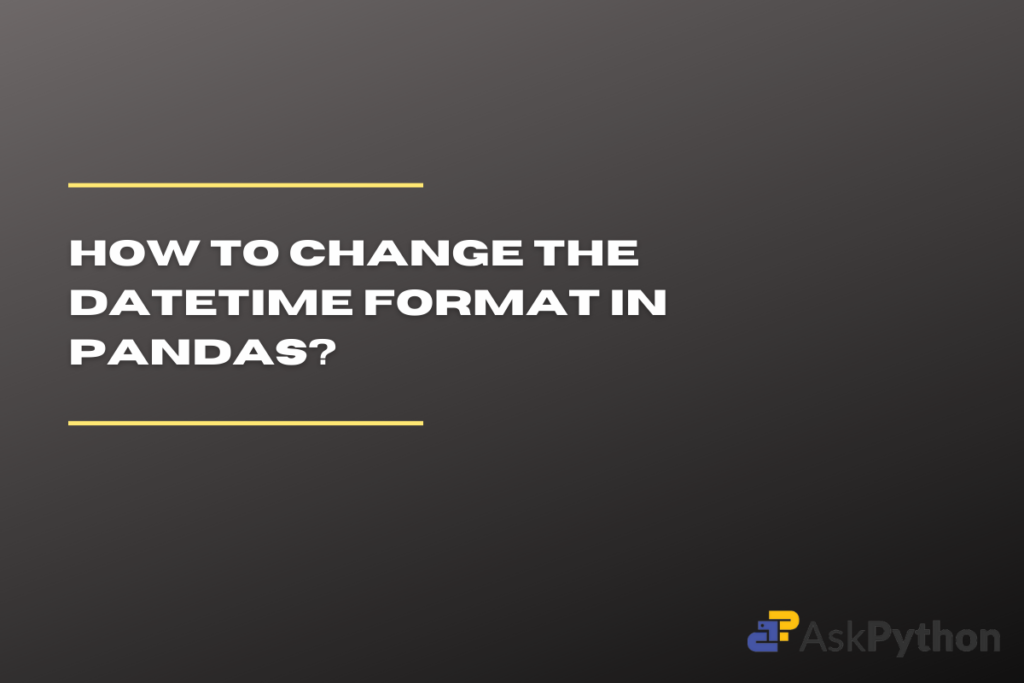# How to Change Datetime Format in PandasThe Pandas package of Python is a great help while working on massive datasets. It facilitates data organization, cleaning, modification, and analysis. Since it supports a wide range of data types, including date, time, and the combination of both – “datetime,” Pandas is regarded as one of the best packages for working with datasets.

The default format of the pandas datetime is set to YYYY-MM-DD, which implies that the year comes first, followed by the month and day values. But what we one requires the format in some other way.

Let us look at the date: 10 April 2000, the default format of datetime will display it as 2000/04/10 but what if one needs to display or store the values in some other format like – 10/04/2000 (DD-MM-YYYY)?

Similarly, as the datetime deals with time as well, the default format of the time is HH-MM-SS which is hours followed by minutes and seconds. What if we need to change the format into seconds followed by minutes and then the hours’ values (SS-MM-HH)?

## Installing and Importing Pandas

This post will guide you on how to do that by formatting the Pandas datetime in your preferred way. As we are working with the Pandas package make sure to install and import the package in your current working IDE before starting with methodologies. To do so run the following lines of code:

```# To install the pandas package
pip install pandas

# To import the pandas package
import pandas as pd
```

The strftime function can be used to change the datetime format in Pandas. For example, to change the default format of YYYY-MM-DD to DD-MM-YYYY, you can use the following code: `x = pd.to_datetime(input); y = x.strftime("%d-%m-%Y")`. This will convert the input datetime value to the desired format.

## Changing Format from YYYY-MM-DD to DD-MM-YYYY

Let’s first create a datetime value using the Pandas to_datetime function, the default format of this function is Year, then month and day values. The data type of the output yield by the function is always ‘object’.

To change the format of the date, we are going to use the `strftime()` – ‘string format time’. This method converts the datetime to a string, so the data type of the output will be a string and not the object. Here the main purpose of using the ‘strftime()’ is to change the format, for that we pass our preferred format as the parameter. The following will help you understand the process more clearly

```input = '10 April 2000'
print("Input: ", input)

x = pd.to_datetime(input)
print("Datetime format: ", x)

y = x.strftime("%d-%m-%Y")
print("Preferred format: ", y)
```

OUTPUT

Similarly, we can also change the format of the date into month first followed by day and year which is MM-DD-YYYY format. Simply pass the format you desired to the `strftime()`

```input = '10 April 2000'
print("Input: ", input)

x = pd.to_datetime(input)
print("Datetime format: ", x)

y = x.strftime("%m-%d-%Y")
print("Preferred format: ", y)
```

OUTPUT

## Changing Format to DD-Month-YYYY

In the above example, the month is represented by the number associated with it. What if we need to print the name of the month instead of the number? The solution remains the same, we use `strftime()`, as it supports many formatting options. Instead of using ‘%m’ pass ‘%b’ for abbreviations of the month and ‘%B’ for the complete name of the month. The following code will help you understand more clearly.

```input = '10 April 2000'
print("Input: ", input)

x = pd.to_datetime(input)
print("Datetime format: ", x)

y = x.strftime("%d-%b-%Y")
print("Month Abbrevation format: ", y)

z = x.strftime("%d-%B-%Y")
print("Month's name format: ", z)
```

OUTPUT

## Changing Time Format from HH:MM:SS to SS:MM:HH

The default format for the time in Pandas datetime is Hours followed by minutes and seconds (HH:MM:SS) To change the format, we use the same strftime() function and pass the preferred format. Note while providing the format for the date we use ‘-‘ between two codes whereas while providing the format of the time we use ‘:’ between two codes.

```input = "13:25:05"
x = pd.to_datetime(input)
print("Datetime format: ", x)

y = x.strftime("%d-%m-%y %S:%M:%H")
print("Preferred format: ", y)
```

OUTPUT